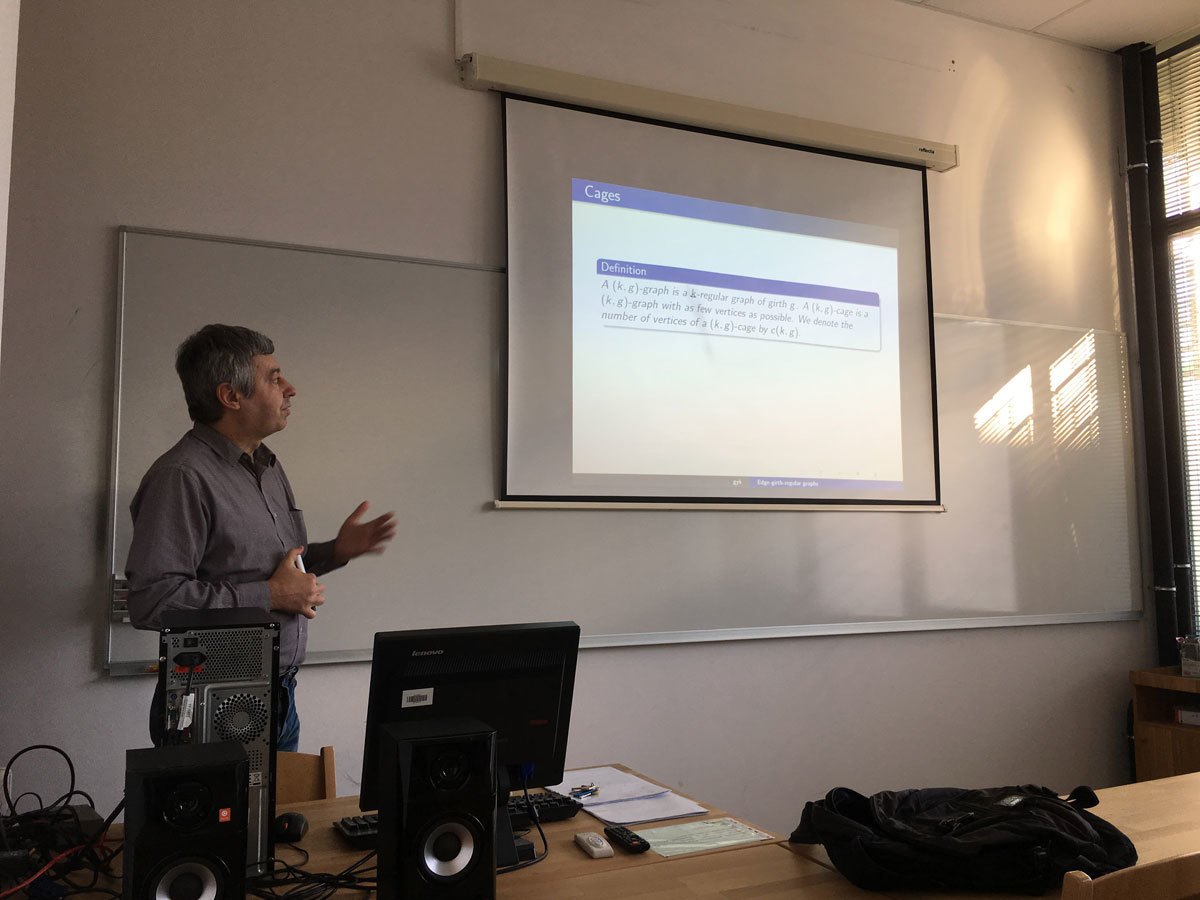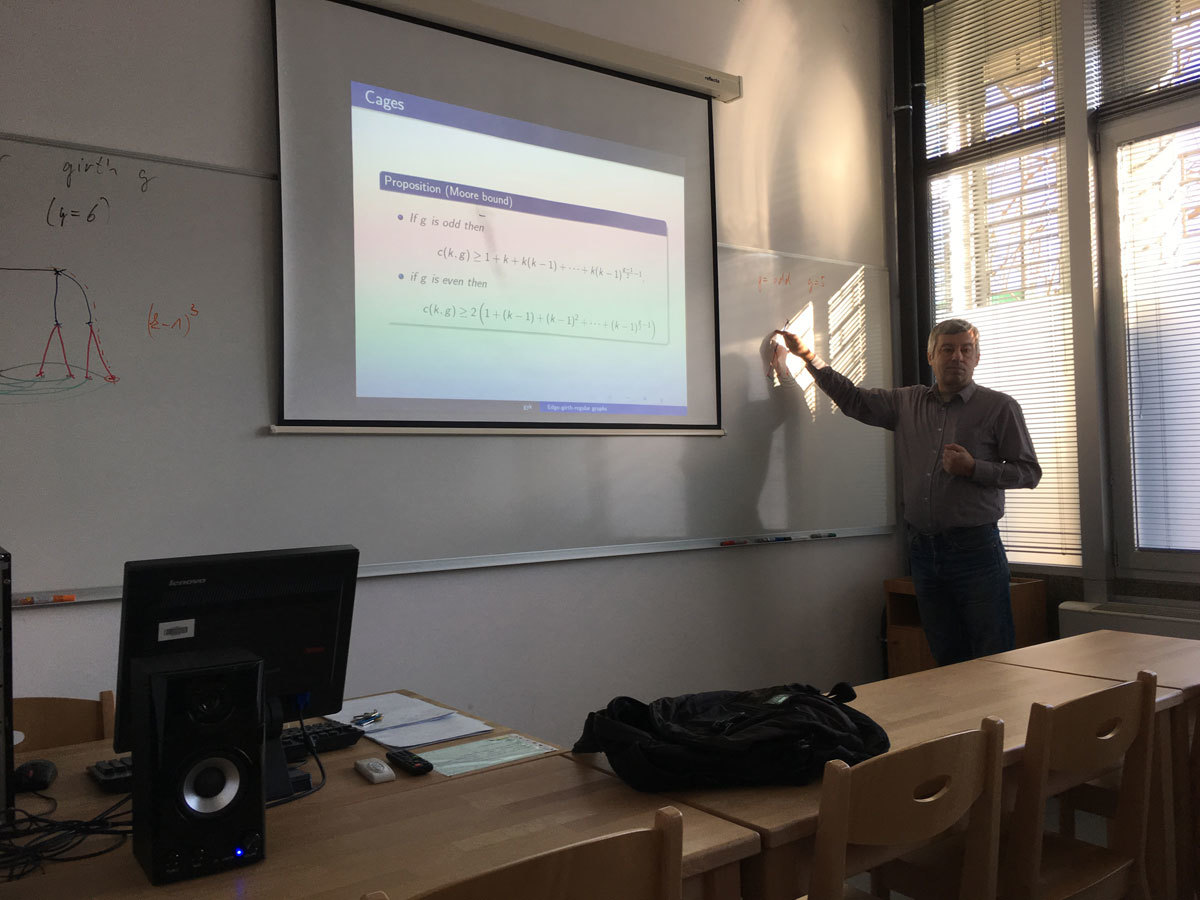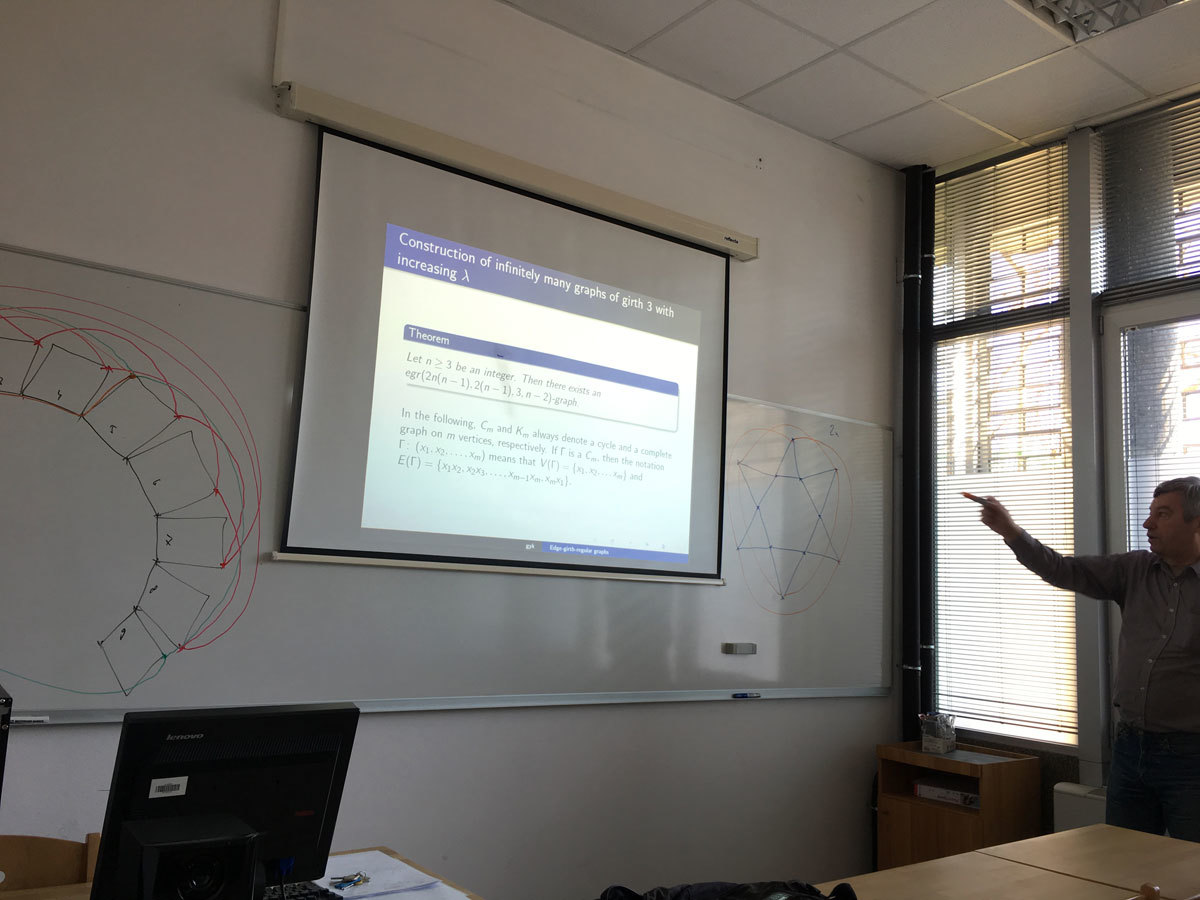Univerza na Primorskem Fakulteta za matematiko, naravoslovje in informacijske tehnologije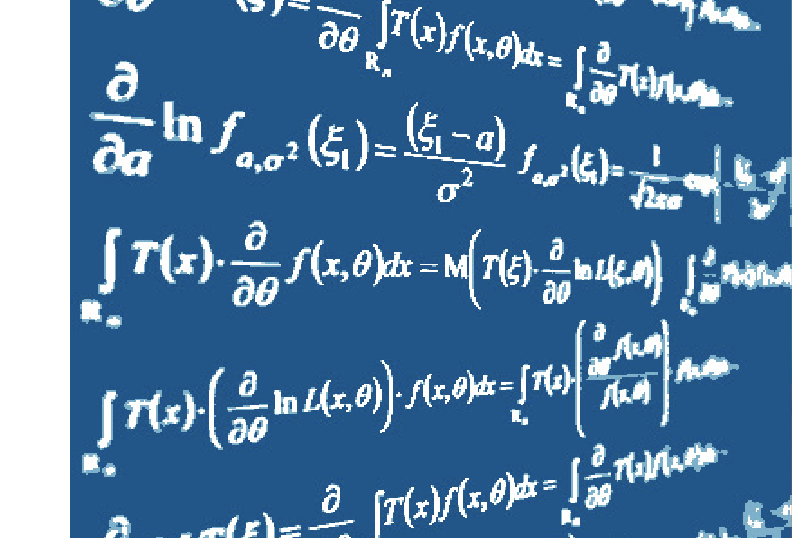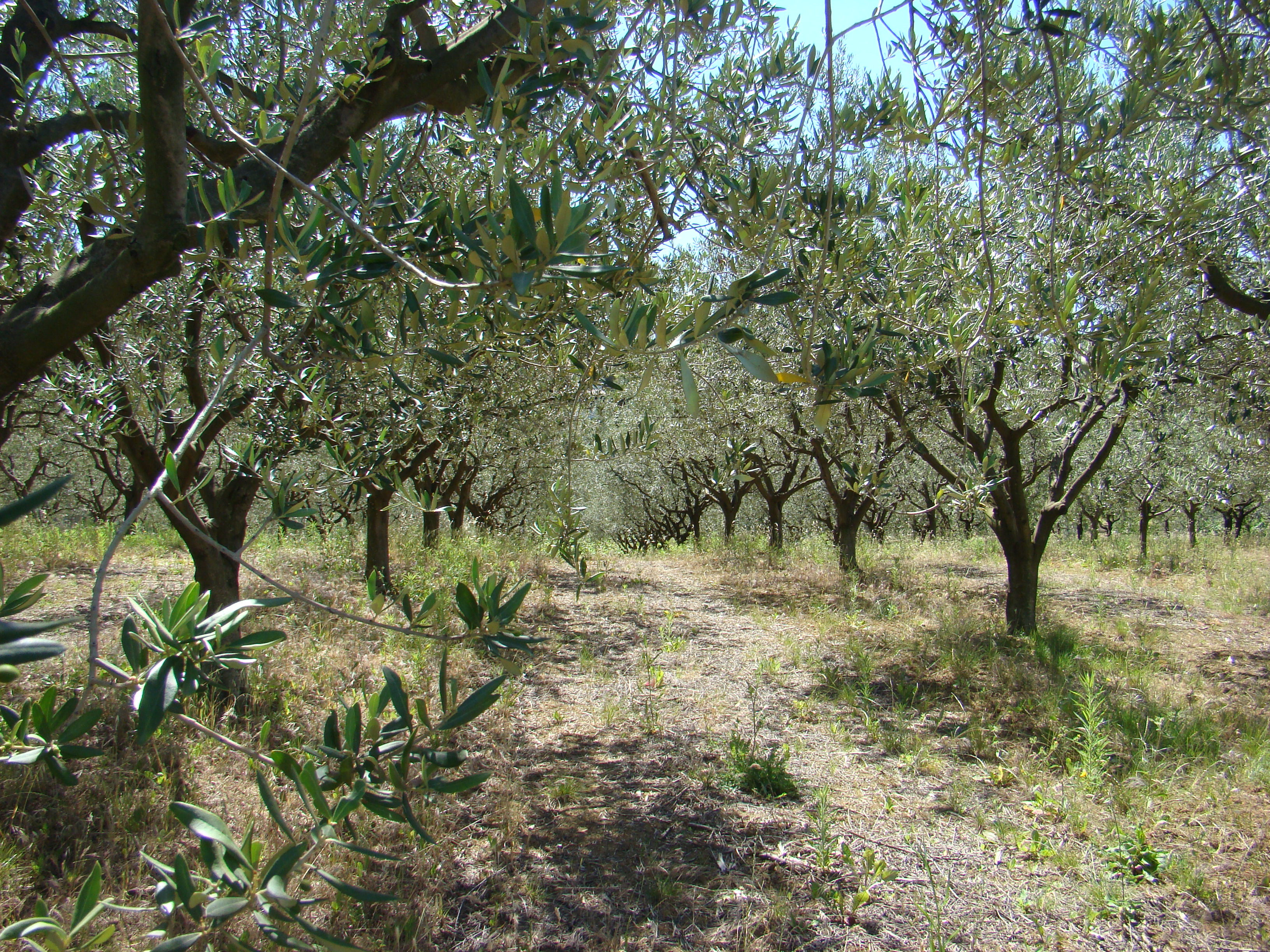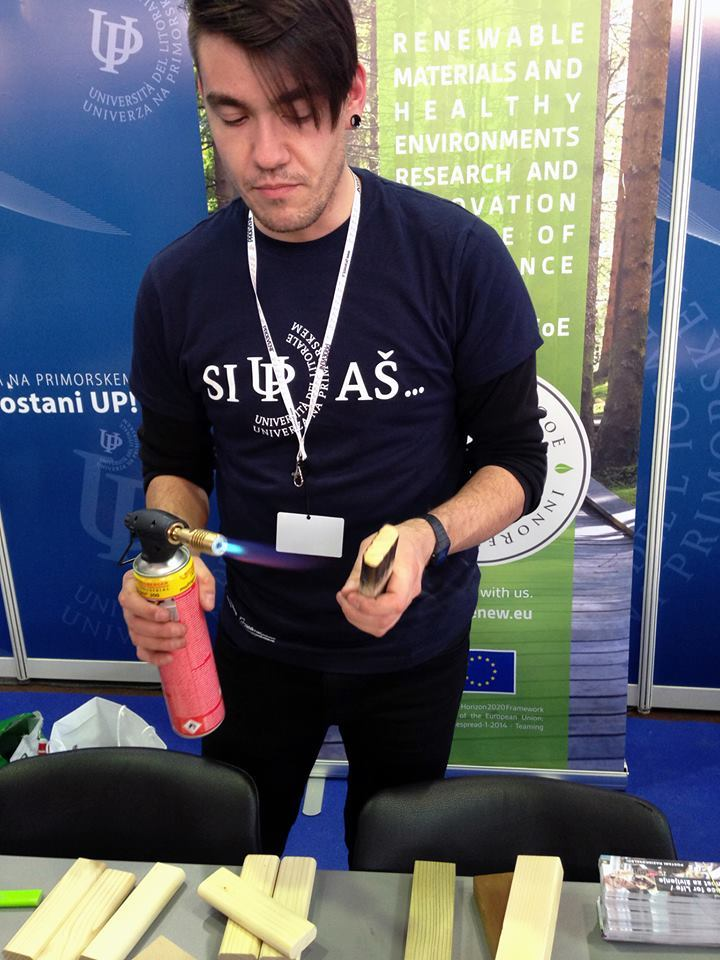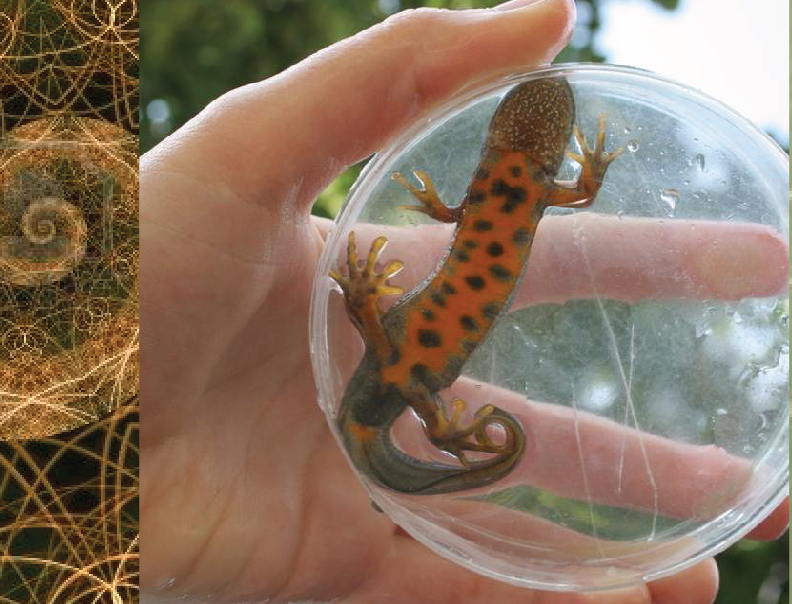SI | EN# Raziskovalni matematični seminar - Arhiv

 2020 2019 2018 2017 2016 2015 2014 2013 2012 2011 2010
 1 2 3 4 5 6 7 8 9 10 11 12
Datum in ura / Date and time: 16.1.17
(10:00-11:00)
Predavalnica / Location: FAMNIT-POŠTA
Predavatelj / Lecturer: Tilen Marc (University of Ljubljana)
Naslov / Title: Minors in partial cubes
Vsebina / Abstract:

We will define and motivate a minor relation in the family of partial cubes. The main topic of the talk will be the forbidden minors characterizations of well established subfamilies. We will present new results on the family of partial cubes that have no forbidden minor isomorphic to a 3-dimensional hypercube minus a vertex. This family plays an important role in understanding bipartite graphs and in establishing a hierarchy among its subfamilies.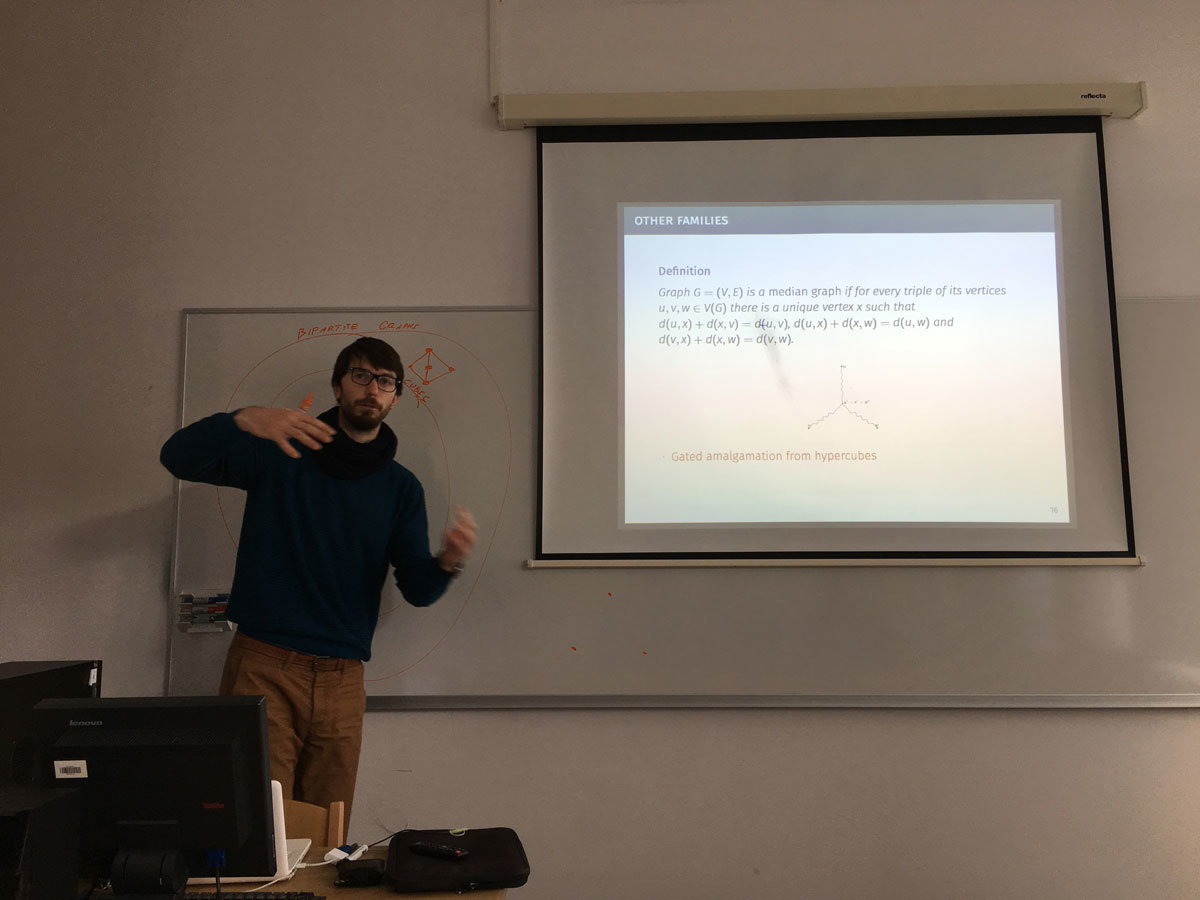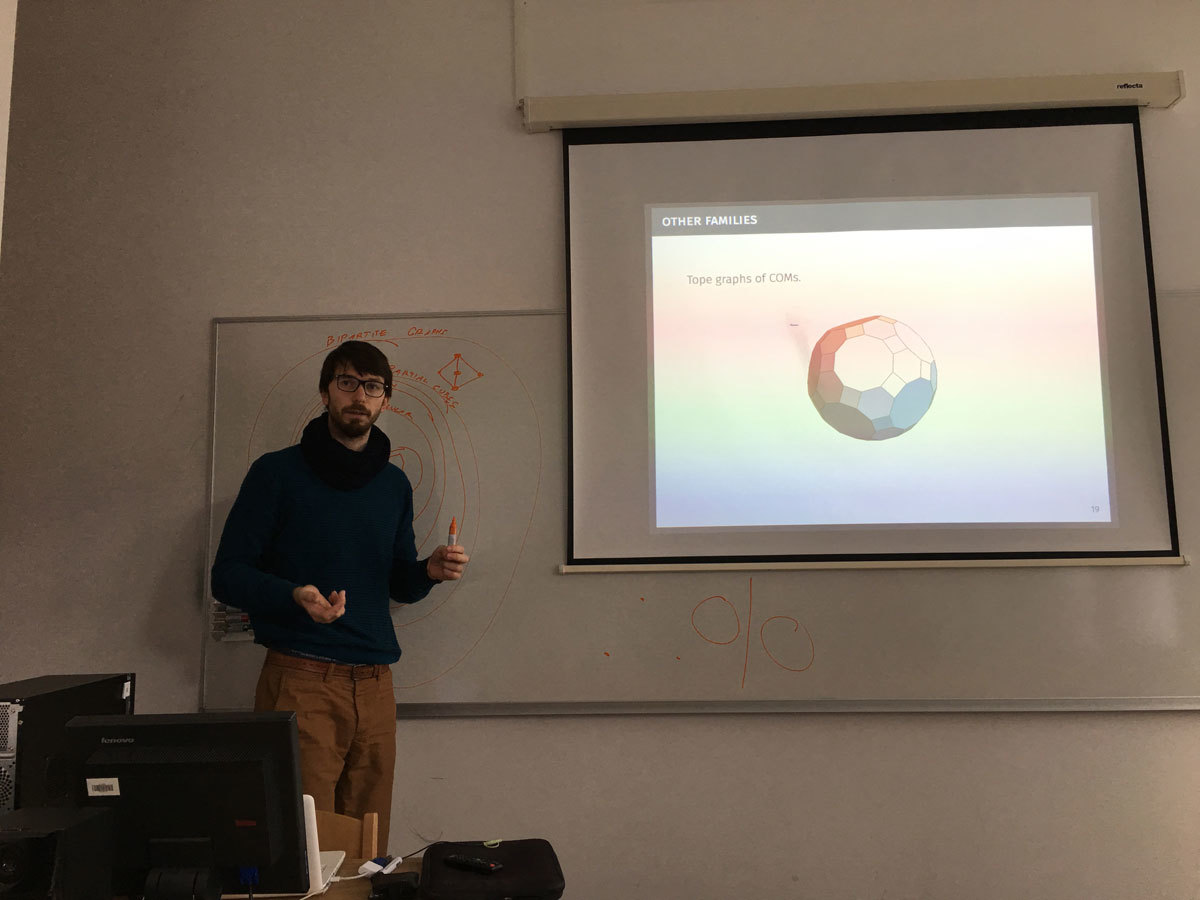Datum in ura / Date and time: 9.1.17
(10:00-11:00)
Predavalnica / Location: FAMNIT-POŠTA
Predavatelj / Lecturer: György Kiss (Eötvös Loránd University)
Naslov / Title: On edge-girth-regular graphs
Vsebina / Abstract:

We consider a new type of regularity we call edge-girth-regularity. An edge-girth-regular $$(v,k,g,\lambda)$$-graph $$G$$ is a $$k$$-regular graph of order $$v$$ and girth $$g$$ in which every edge is contained in $$\lambda$$ distinct $$g$$-cycles. This concept is a generalization of the well-known $$(v,k,\lambda)$$-edge-regular graphs (that count the number of triangles) and appears in several related problems such as Moore graphs and Cage and Degree/Diameter Problems. All edge- and arc-transitive graphs are edge-girth-regular as well. We derive a number of basic properties of edge-girth-regular graphs, systematically consider cubic and tetravalent graphs from this class, and introduce several constructions that produce infinite families of edge-girth-regular graphs. We also exhibit several surprising connections to regular embeddings of graphs in orientable surfaces.

Joint work with Robert Jajcay and Štefko Miklavič.